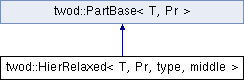# twod::HierRelaxed< T, Pr, type, middle > Class Template Reference

implements the hierarchical relaxed heuristic and some variants. More...

`#include <twod/rec_bisect_relaxed.hpp>`

Inheritance diagram for twod::HierRelaxed< T, Pr, type, middle >:## Detailed Description

### template<typename T, typename Pr, int type = 0, bool middle = false> class twod::HierRelaxed< T, Pr, type, middle >

implements the hierarchical relaxed heuristic and some variants.

Parameters:
 T is the data type of prefix sum array. Pr is the 2D prefix sum array. type is the recursive bisection strategy type. Strategy types are LOAD, DIST, VERT_ALT, HOR_ALT

List of all members.

## Public Member Functions

virtual T part (int procCount, const Pr &prefixSumArray, util::RectList< T, Pr > &parts)
Applies a 2d partitioning algorithm to a given 2d prefixSumArray .
virtual ~HierRelaxed ()

## Static Public Attributes

static const int LOAD = 0
static const int DIST = 1
Cut to minimize side-to-side distance.
static const int VERT_ALT = 2
start vertically and alternate between horizontal
static const int HOR_ALT = 3
start horizontally and alternate between vertical

## Static Private Member Functions

static T rec_bisect_2d_internal (int procCount, const Pr &prefixSumArray, util::RectList< T, Pr > &parts, util::rectangle r, bool orient)

## Constructor & Destructor Documentation

template<typename T , typename Pr , int type = 0, bool middle = false>
 virtual twod::HierRelaxed< T, Pr, type, middle >::~HierRelaxed ( ) ` [inline, virtual]`

## Member Function Documentation

template<typename T , typename Pr , int type, bool middle>
 T twod::HierRelaxed< T, Pr, type, middle >::part ( int procCount, const Pr & prefixSumArray, util::RectList< T, Pr > & parts ) ` [virtual]`

Applies a 2d partitioning algorithm to a given 2d prefixSumArray .

Parameters:
 [in] procCount is the number of processors [in] prefixSumArray first column and first row consists of zero only. But the borders of rectangles in rect_list never touch this area (index 0 in row or column) [out] parts must be empty before calling this function. It will contain the partition after the function return
Returns:

Implements twod::PartBase< T, Pr >.

template<typename T , typename Pr , int type, bool middle>
 T twod::HierRelaxed< T, Pr, type, middle >::rec_bisect_2d_internal ( int procCount, const Pr & prefixSumArray, util::RectList< T, Pr > & parts, util::rectangle r, bool orient ) ` [static, private]`

## Member Data Documentation

template<typename T , typename Pr , int type = 0, bool middle = false>
 const int twod::HierRelaxed< T, Pr, type, middle >::DIST = 1` [static]`

Cut to minimize side-to-side distance.

template<typename T , typename Pr , int type = 0, bool middle = false>
 const int twod::HierRelaxed< T, Pr, type, middle >::HOR_ALT = 3` [static]`

start horizontally and alternate between vertical

template<typename T , typename Pr , int type = 0, bool middle = false>
 const int twod::HierRelaxed< T, Pr, type, middle >::LOAD = 0` [static]`

 const int twod::HierRelaxed< T, Pr, type, middle >::VERT_ALT = 2` [static]`